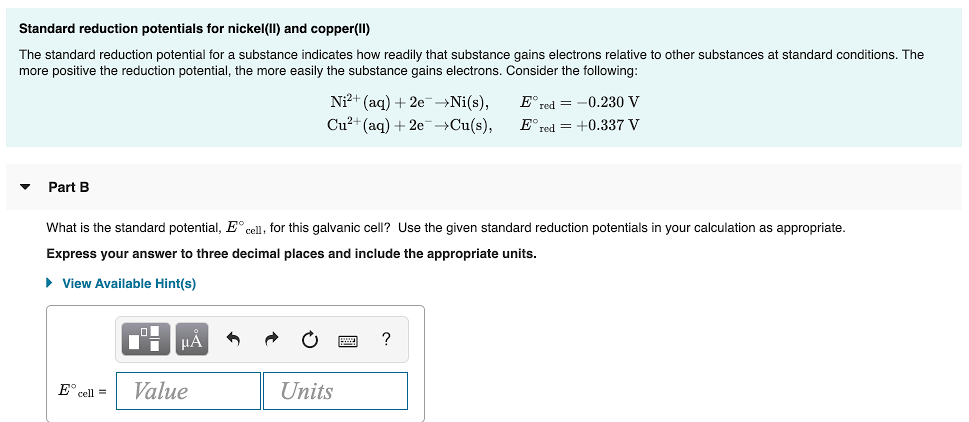# The standard reduction potential for a substance indicates how readily that substance gains electrons relative to other substances at standard conditions. The more positive the reduction potential, the more easily the substance gains electrons. Consider the following: N2^2+(aq) + 2e^- → Ni(s), E°red = -0.230 V Cu^2+(aq) + 2e^- → Cu(s), E°red = +0.337 V What is the standard potential, E° cell, for this galvanic cell? Use the given standard reduction potentials in your calculation as appropriate. Express your answer to three decimal places and include the appropriate units.